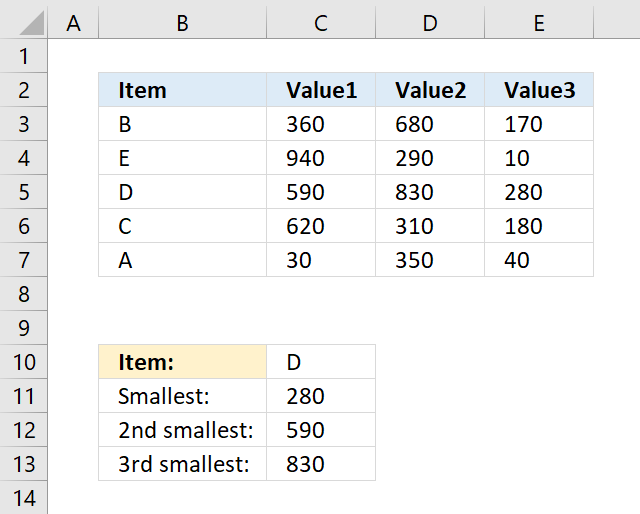Author: Oscar Cronquist Article last updated on November 15, 2018The array formula in cell C11 gets 3 values in one fetch, the INDEX function allows you to do that if you enter 0 (zero) in the row or column argument. The SMALL function then calculates the k-th smallest value of these three values.

=SMALL(INDEX(\$C\$3:\$E\$7, MATCH(\$C\$10, \$B\$3:\$B\$7, 0), 0), ROWS(\$A\$1:A1))

To enter an array formula, type the formula in a cell then press and hold CTRL + SHIFT simultaneously, now press Enter once. Release all keys.

The formula bar now shows the formula with a beginning and ending curly bracket telling you that you entered the formula successfully. Don't enter the curly brackets yourself.

Allow me to explain the formula, the MATCH function returns the location of a specified value in an array och cell range.

MATCH(\$C\$10, \$B\$3:\$B\$7, 0)

becomes

MATCH("D",{"B";"E";"D";"C";"A"},0)

Value D is found in position 3 in this array: {"B";"E";"D";"C";"A"}

INDEX(\$C\$3:\$E\$7, MATCH(\$C\$10, \$B\$3:\$B\$7, 0), 0)

becomes

INDEX(\$C\$3:\$E\$7, 3, 0)

The INDEX function then returns all values on relative row 3 in this cell range \$C\$3:\$E\$7: {590, 830, 280}

SMALL(INDEX(\$C\$3:\$E\$7, MATCH(\$C\$10, \$B\$3:\$B\$7, 0), 0), ROWS(\$A\$1:A1))

becomes

SMALL({590, 830, 280}, 1)

and returns 280 in cell C11.

You can also use the SMALL function to match multiple values, make sure you read this post: 5 easy ways to VLOOKUP and return multiple values